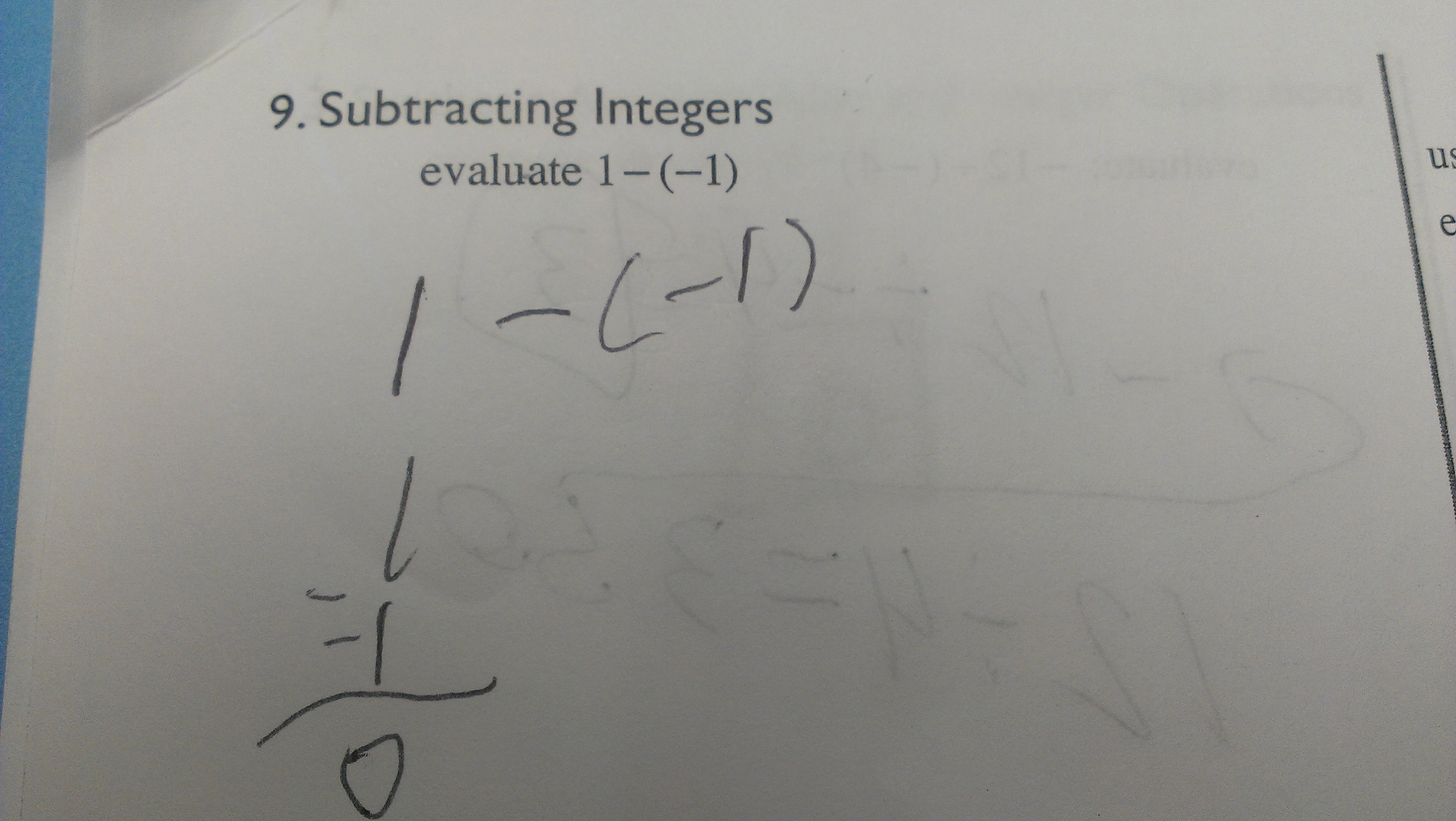Categories

# 1 – (-1) = …Where does this mistake come from? I mean, the kid knows that 1 – 1 = 0, right? So does the kid think that 1 – (-1) = 0 too, or does the kid misconstrue this as 1 – 1? What’s your theory?

Thanks to Chris Robinson for the work sample.

## 3 replies on “1 – (-1) = …”

Emmettsays:

I bet the student does know that 1-1=0, but understanding that this fact means that 1-(-1) cannot = 0 requires a much higher level of reasoning. My guess would be that the student just saw a minus sign and subtracted. How do we fix it? Probably just more practice with negative numbers to try to develop the concept of what it means to subtract a negative number.

It does seems like the kid wasn’t thinking about what it *meant* to subtract a negative number. It seems like setting up the long form of traditional subtraction kicked in “now I follow those rules from 2nd grade.” This reminds me of why I ask my students not to use cross multiplication and things like that — they try to memorize these steps, but then set them up in a weird way and don’t really pay attention to the meaning of what they’re doing. I imagine that’s what made this student look at the two ‘-‘ symbols and just remember to subtract. I would think that discussing specifically what each part of that set-up means might help, or encouraging them to abandon it for the time-being and going back when there’s more understanding, if it’s just causing problems.

Juliesays:

This kid thinks that 1 – (-1) = 1 – 1. He or she does not realize that there is a difference between those two equations. The kid is not thinking about the problem as 1 minus negative 1, instead he or she sees it as 1-1, which is how the kid got the answer 0. The negative symbol just seems like 2 minus signs probably because the student does not understand what (-1) means in a equation.